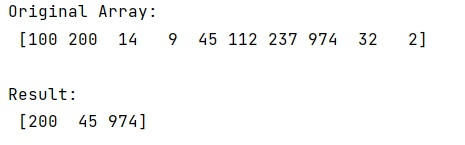# How to get the values from a NumPy array using multiple indices?

Given a NumPy array, we have to get the values it using multiple indices.
Submitted by Pranit Sharma, on February 17, 2023

NumPy is an abbreviated form of Numerical Python. It is used for different types of scientific operations in python. Numpy is a vast library in python which is used for almost every kind of scientific or mathematical operation. It is itself an array which is a collection of various methods and functions for processing the arrays.

## Getting the values from a NumPy array using multiple indices

Suppose that we are given a numpy array that contains some numerical values. We need to find a way to get the multiple values from this array by index. For example, if we are given an array of 10 elements, we need to find the values of this array at positions 1,4, and 7.

For this purpose, we will use a list of indices inside [ ] on our numpy array so that it reads all the indices one by one and return their corresponding values.

Let us understand with the help of an example,

## Python code to get the values from a NumPy array using multiple indices

```# Import numpy
import numpy as np

# Creating a numpy array
arr = np.array([100, 200, 14, 9, 45, 112, 237, 974, 32, 2])

# Display original array
print("Original Array:\n",arr,"\n")

# Getting values using multiple indices
res = arr[[1,4,7]]

# Display Result
print("Result:\n",res)
```

Output: